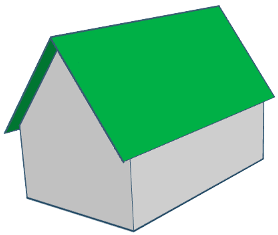# How to calculate the roof area from house area and pitch- Roof incline (pitch US )
- House surface (plus the area with which the roof extends outside the house - it can be calculated by multiplying the extension with the perimeter of the house).

#### How to calculate Roof surface

For a roof incline of (pitch x/12):
/12
You dont know the pitch?
Use our calculators
We find the slope factor from pitch:
Slope factor = (hypothenuse of rise 4 and run 12) / 12
We find the hypothenuse using Pythagorean theorem:
hypothenuse² = 4² + 12² = 160
Hypothenuse is therefor squared root of 160:
hypothenuse = root of 160 = 12.65
Slope factor is hypothenuse divided by 12:
slope factor = 12.65 / 12 = 1.0541
Surface of the roof = house surface x slope factor:
%
x 1.0541 = 210.82
Total roof surface = 50

Warning!
This formula for calculating the roof surface produces approximate results! The resulted surface area is similar with that of a gabled roof, but other roof shapes might have a very different surface area!
One advantage of this formula is that it can be used as an approximation for houses with unique shapes or with complicated roofs - we can use it to obtain a very general idea about the roof surface.
Please check our roof shape calculators - if we have a calculator for your roof shape, it is going to produce pretty accurate results. .